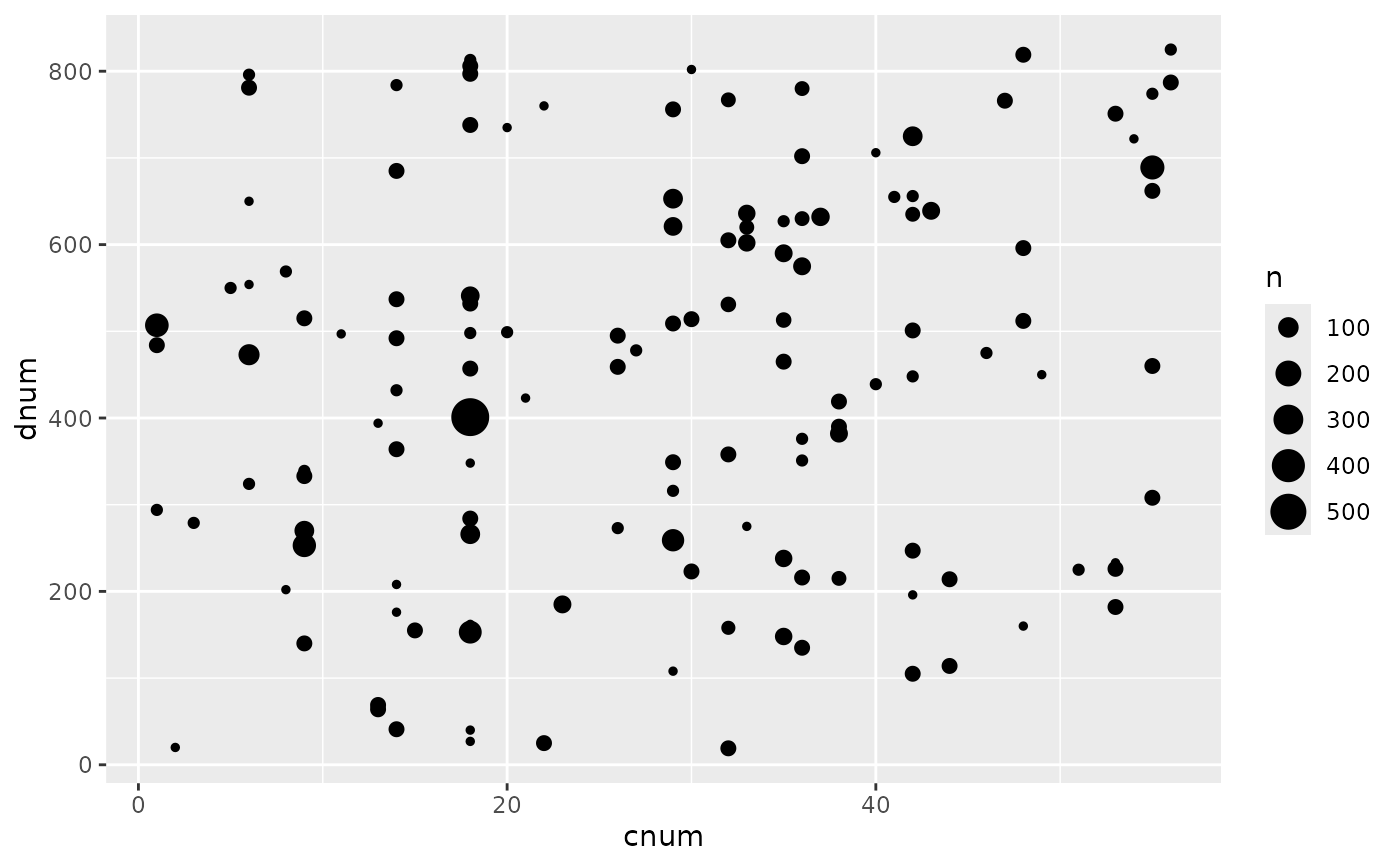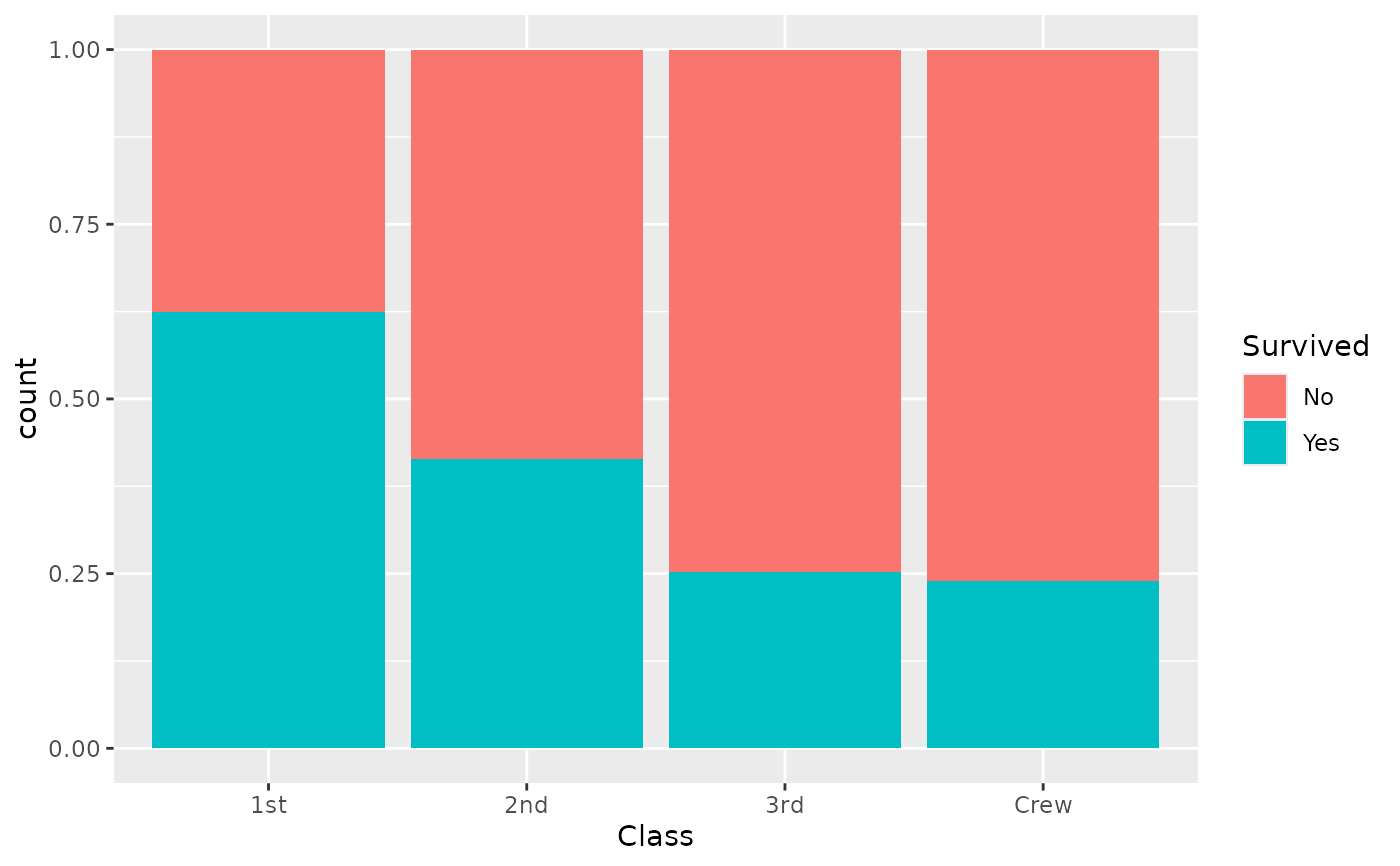A function to facilitate ggplot2 graphs using a survey object. It will initiate a ggplot and map survey weights to the corresponding aesthetic.

ggsurvey(design = NULL, mapping = NULL, ...)

## Arguments

design

A survey design object, usually created with survey::svydesign()

mapping

Default list of aesthetic mappings to use for plot, to be created with ggplot2::aes().

...

Other arguments passed on to methods. Not currently used.

## Value

A ggplot2 plot.

## Details

Graphs will be correct as long as only weights are required to compute the graph. However, statistic or geometry requiring correct variance computation (like ggplot2::geom_smooth()) will be statistically incorrect.

## Examples

data(api, package = "survey")
dstrat <- survey::svydesign(
id = ~1, strata = ~stype,
weights = ~pw, data = apistrat,
fpc = ~fpc
)
ggsurvey(dstrat) +
ggplot2::aes(x = cnum, y = dnum) +
ggplot2::geom_count()d <- as.data.frame(Titanic)
dw <- survey::svydesign(ids = ~1, weights = ~Freq, data = d)
ggsurvey(dw) +
ggplot2::aes(x = Class, fill = Survived) +
ggplot2::geom_bar(position = "fill")